####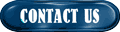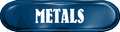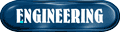####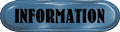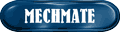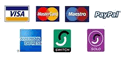###How to calculate a dimensional point in an arc or radius

 Sometimes it may be necessary, and I have been asked on several occasions the question of how does one calculate any point along an arc - usually for reasons of machining a sphere and such like. This short paper will take you through the process of working out any point over the surface of the arc/radius/sphere and then how to incorporate the calculation into something that either a CNC, PC or DRO user can use to locate or produce a feature or full sphere in a work-piece.The job that I had to perform was to machine a perfect radial ball seat in a number of castings that would allow a smooth interface between a ball fitting and the cast housing.

Luckily the job was one of prototype development rather than a breakdown, because upon thinking about the task I thought that the math would be fairly simple - and it is, now that I know how to do it - but at the time I ended up asking several university lecturers for an answer - but to no avail. In the end it took me many hours sat with an old engineering handbook to come to a suitable calculation that I could use that my CNC (Fanuc) machine would understand.

(At the time, all programming was performed 'long hand' before we had CadCAM software)

The principal of the calculation relies on working out the cord of a radius as follows:

'C' is the cord dimension.

'H' is the layer height*.

*Layer height is taken as an incremental position from Z0.0. So that in traditional CNC programming where a profile is 'layered' into slices of any thickness to build the 3d shape, 'H' would be taken a zero height at the bottom of the radius.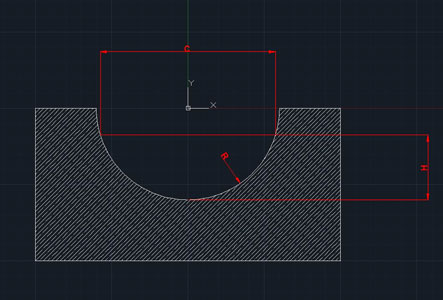### CALCULATION

This is the formula showing all three possible combinations of working. So if you need to know the height from Z0 of a point of a known radius then you can use the 'H=' formula as thus:

Height= Radius minus 0.5 times the square root of 4 times the radius squared minus the projected diameter of the point on the radius (this can be either a layer level or in the case of a hole or detail then in this case it is just a dimensional point in workspace)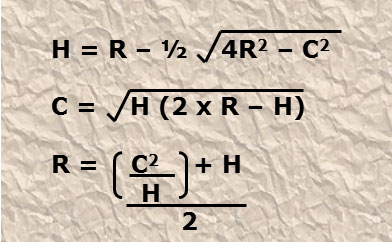### THE CODE

Now to put this into CNC code. In this example I’m using FANUC with G40 G90 and G17 programmed before the machining routine to cancel all offsets and work in ABS mode.

So, here I’m using variables:

#V4 = Axis movement (ABS Z distance).

#V9 = The layer or height of the detail.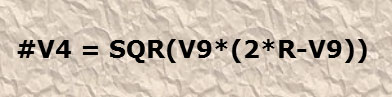Here is the compressed version of the calculations used above into this plain English piece of code:

#V4 (or variable 4) is equal to the square root of the layer or height from Z0 of the detail (#V9) times 2 times the radius minus the height from Z0.

The parenthesis must be used in FANUC exactly as shown in order for the processor to calculate the expressions correctly. (assuming the machine is Fanuc Macro command capable.)

PUT IT TO USE

This is the code directly programmed into the machining centre. It takes full advantage of the macro capability of FANUC to hold variables that keep track of layer, radius and general program position.

So from the top, we set up the machine using the usual G80 G90, we also run in G8 mode to add quick execution commands. We use S5000 to run at 5000RPM and F700 for 700mm/Min feed rate. (yes unfortunately we are using metric in this case).

The next block sets up the working variables including that of manual tool radius compensation - in this case we do not use auto compensation as the controller can get confused as we circle the datum point of 0,0 whilst taking cuts (or layers) of 0.2mm per cut.

We now pass the variables to #Boreloop to first machine a parallel bore 17mm deep before moving to #Radloop whereby we continually check the 'Z' axis encoder output to test if we reach a '0.0' condition (the centre point of the radius).

You can now see that the variables V9R9 keep track of our depth and position as we machine deeper into the material, each pass or layer we recalculate all of the axis positions by using variable V70 (the output of V70 will always have minus 10.0mm taken from its result to compensate for the cutter tip radius). All variables pass to a PRINT command to display to the operator the progress of the job. The important part here is that V70=SQR(V9*(V90))-10.0 which calculates our position on the radius per layer as we move downwards through the material.

We then perform two checks on both the math and the encoder axis position to determine if we have reached the bottom and central point of our sphere (I like to belt and braces just in-case of any error!)

Once we've reached the end of the profile we move to #End which terminates the routines and sets our cutter back above the job before performing a full stop with M5M9M0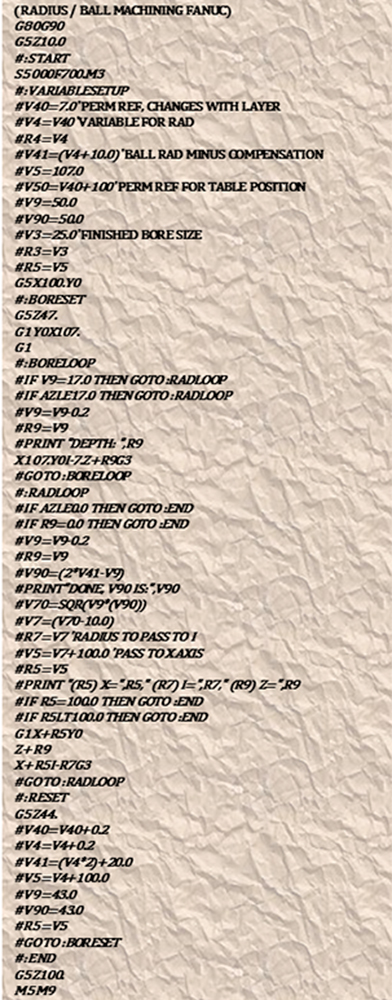Although the code is written specifically for a FANUC based system, the general math and process can be reproduced on virtually any CNC capable of 'on-the-fly calculation' (most machines produced after 1993-1994)

-Thomas Jones 2017